# ERRATA

Please email me to report any problems, small or big, to john1@maxvalue.com. Thanks for reading the book!

Decision Analysis for Petroleum Exploration, 3.0.x Edition

Error persisting in several revisions:

You may want to check further down the list after your edition. Posted revisions are for the latest edition where someone noticed the error.

To facilitate users with various editions, section numbers will replace page numbers.

The 3.1 edition (est. 2020) will lock-down page numbers and section references.

Upcoming nomenclature changes:

Upon checking several authoritative sources (e.g., Wikipedia, Wolfram Research), it seems only half the world uses lower-case for distribution abbreviations (Wikipedia authors have used mostly, but not always, lower-case). With no clear choice, I've decided to start capitalizing (which I prefer). Capitalized abbreviations are much more visible in text. So pdf's will become PDFs, cdf's will become CDFs, etc.

Here are two other forthcoming changes:

Since perhaps high school, I've used "CDF" believing the "D" stood for "density." Either the meaning of CDF changed at some point or I've always had it wrong.

Wikipedia has a disambiguation page saying that "cumulative density function" is self-contradictory. https://en.wikipedia.org/wiki/Cumulative_density_function

1. The present and widely-accepted meaning of CDF is cumulative distribution function. This applies to both continuous, discrete, and mixed-type distributions.

2. Similarly, I have been using "rcdf" to represent reverse cumulative density function. Some prominent software packages (still) use reverse for labeling frequency distributions. However, especially with density functions, the more-accepted term appears to be complementary cumulative distribution function (CCDF). I'll be using that and, similarly, CCFD to represent complementary cumulative frequency distribution.

Other upcoming corrections:

3.0.6 version released Mar 2015

2.015 Probability Density Function

The second, CDF, chart is mis-labeled. This is a "less than chart." The y-axis should read the "Probability of being Less Than".

2.016 Probability Mass Function

The "Cumulative Less-Than Probability Mass Function" figure is mislabeled. Because the large dots are at the top of the up bars, this is a cumulative-less-than-or-equal-to probability mass function.
(Next edition, I'm moving the dots down.)

2.316 Beta Distribution

Apparently, this kernel form is more-popular (with, e.g., @RISK, Crystal Ball):And the normalizing factor denominator is: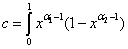The distribution now has these simple properties (Thanks to Palisade Corp. for the excellent @RISK documentation):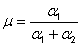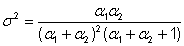2.605 Working with Low Probability Events

The last part of the caption for the first figure reads:
while this may look there is significant probability that Strain > Failure, the actual probability is much lower than one might expect.

while this may look like there is significant probability that Strain > Failure, the actual probability is much lower than one might expect.

6.320. Typical Value of Information Problem (p. 441; the error is on p. 445):

In the paragraph above the last tree, "EMV is \$60 million (80-20) higher with the Do Pilot Alternative." should read "EMV is \$57 million (77-20) higher with the Do Pilot Alternative."

The table, below, details the VII calculation.

3.0.5 version released December 2014

2.017. Frequency distribution
The Retrieval Code for the corrected Excel-produced frequency distribution chart is
uqueqk

5.214. Sensitivity Chart

The 100% W.I. line starts at DHC (~\$40 million) at Ps = 0 and is the Discovery Value (\$175 million) at Ps = 1.

Index entry "ME2"
These are supposed to be "Excel" (the dummy name was used in a search-and-replace operation and not restored).

3.0.4 version released August 2014.

2.060. Correlation Coefficient

All three equations for σxy, σxx, and σyy : The summation terms (Σ's) should all be preceded by 1/n.

3.0.2 version released April 2014.

2.231. Bayes by Probability Tree
In the subsection, "Conditional probabilities on the tree" subsection. The first three joint probabilities at the top portion should be switched with the bottom three:
As examples, P("DHI" | HC) = .50 and P("DHI" | no HC) = .10.

2.701. The reader can ignore the obsolete references to Table 3.1 and Chapter 6 that can be ignored. An expanded discussion of the same example (except monetary outcomes were multiplied by 100) is available with Retrieval Code vrza.

2.720. Again there is a reference to Table 3.1 and to original chapter numbers. Some of the properties of EV are discussed in 2.130 Algebraic Properties of EV."

2.731. Assessment Challenges. This is mostly about subjective assessments and biases. Please see 2.354 Subjective vs. Objective Probabilities and 2.730 Cognitive and Motivational Biases.

2.733. Risking Petroleum Exploration. This section has been dropped. For judgments see 2.354 Subjective vs. Objective Probabilities and 2.730 Cognitive and Motivational Biases. We have seen companies use multi-criteria scoring methods; we advise that rough economic evaluations be attempted instead.

2.735. Resource Distributions. There are only Figures 3 and 4 in this topic.

2.1 Edition and Earlier

Decision Analysis for Petroleum Exploration, Second Edition and Earlier

Special thanks to Larry Killion, PE, J.D., BHP Billiton Petroleum, who noticed and reported quite a few typographical errors.

Some of the recent and more-important revisions are listed immediately below:

#### Page 1  [I can't believe this error on the first page!] (prior to the 2005 printing)

The text box in the left margin truncates the last word and should read: "Petroleum exploration is a classic case of decision-making under uncertainty."

#### Page 16, Missing Figure 2.3 at bottom of page (prior to the 2007 printing):

(Somehow, the computer didn't print the figure.)#### Page 21, Table 2.1 7th row  (2nd edition, 2009 printing and earlier)

• Total New Revenues:
• Prospect A:  \$485,500 should be \$485,450.
• Prospect B:  \$589,000 should be \$589,282.

#### Pages 29, 46, 55. Appendix A references (2nd edition, 2007 printing and earlier):

The "Appendix A" references direct the 1st Edition reader to present value tables. We now have Excel and financial calculators, so the appendix was dropped in the 2nd Edition.

#### Page 25 and pages 568-9.  PV Discount Rate and Decision Policy Update:  Current thinking.

I have long maintained that EMV of a project, properly evaluated, provided a measure of the value added to the corporation. In prior efforts to model project and company value from the shareholder's perspective, a persistent problem has been the inconsistency with the shareholder's time value of money and the typical capitalization rates inferred from stock prices (papers HEES3 and HEES5). A fundamental premise in the decision analysis approach is that the PV discount rate should represent only time value of money, and that risking should be done with probabilities.

In developing an example for the latest in a series of papers (HEES6), I was working to reconcile EMV per share versus share price. Using Value Line Investment Survey data for nine oil and gas producers, I was finding that share price was typically about half of the PV per share of forecast free cash flow. I used a multiplicative factor to represent an additional market value discount (MVD) factor [which I formerly called 'discount to market capitalization' (DMC) factor]. Factoring NPVs for risk is a time-honored adjusting method for people buying and selling cash-producing assets (such as petroleum producing properties). While I'm unaware of any theory suggesting this is the one, correct calculation method, this MVD factor provides a means to adjust values downward without resorting to an excessively-high PV discount rate.

My current (as of Sep. 2006) thinking is:

• EMV_of a project or the company should be based upon modeling the project's contribution to the company's free cash flow.
• EMV_of a project, properly evaluated, measures incremental EMV of the company.
• EMV_of the company (calculated from free cash flow) ´MVD factor = market capitalization of the company.
• Shareholder value added by a project equals the project EMV times the MVD factor.
• Replacing EMV with certainty equivalent (CE, page 204) will be somewhat better. This will incorporate investor's risk aversion as to the outcome levels. Use the exponential utility function and scale-up the typical investor's risk tolerance coefficient to the company level. Normally, this will be a minor adjustment.
• The "optimizer's curse" bias (see inset) needs to be estimated and adjusted-out.

In my opinion, the appropriate free cash flow consists of the cash amounts that can be aggressively withdrawn from the company, without particular regard to maintaining the business. This free cash flow definition is different from the customary one that assumes that business assets are maintained or replaced.

 Yet another detail affects our use of the EMV decision rule: The "optimizer's curse." Tim Nieman brought this to my attention recently, and I've referenced the source paper, summarized the concept, and prepared some demonstration calculations into Tip 113 (which links to a PDF file). Investment candidates with slightly-positive EMVs should not be approved. Forecasts of a project's value-added will probably be too high. Even if our evaluations are unbiased, random errors will introduce an optimistic bias when we select the better projects. Bayesian calculations are needed to adjust-out this bias.

If you have any comments or suggestions as to a better approach, please contact me:I hope to wrap-up this topic in yet one more paper or article. It will be mainly about the optimizer's curse and reversing this bias in a consistent approach to capital investment decisions and portfolio optimization.

#### Page 53, "10%" missing (2nd edition, 2007 printing and earlier):

Near the bottom,

"Finally, the gain per year in excess of making an earnings rate of   is computed as ... " should read

"Finally, the gain per year in excess of making an earnings rate of 10% is computed as ... "

#### Page 77, last formula (2nd edition, 2007 printing and earlier)   another try 16:46: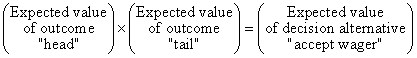#### Page 103, Table reference (2nd edition, 2007 printing and earlier):

The last paragraph, "Table 3.6 shows the ratio calculations." Because the table wasn't numbered in the 2nd edition, this should read

The table on page 104 shows the ratio calculations."

#### Page 185, Reference to Equation 5.1 (2nd edition, 2007 printing and earlier):

About the middle of the page, "Equation 5.1 states ..." should read "The equation above states ..."

#### Page 209, middle of page, figure reference (prior to the 2005 printing).

"[such as Figure 5.1 (c)]" should be "[such as Figure 5.5 (c)]".

#### Page 227, "1:10" odds (2nd edition, 2007 printing and earlier):

The second paragraph ends with "probability of a discovery is 1/10 or 0.10." and should instead read "probability of a discovery is 1/11 or 0.0909."

Page 232, P(A+B+C) formula (prior to the 2007 printing).

The formula is missing the P(C) term and should read:

P(A+B+C) = P(A) +P(B) +P(C) -P(AB) -P(AC) -P(BC) +P(ABC)

Page 256, Frequency Histogram: Wrong figure printed  (2nd edition, 2007 printing and earlier):

Table 6.6 has a typo in the middle row. The frequency of the wells with thicknesses 110-150 should be 5, not 6.

Figures 6.12 and 6.13 are the same.  Figure 6.12 (update version below) is supposed to have the y-axis in 'Number of wells having thickness values in each range.' That is, the y-axis should be units that are number of 'counts' in each histogram bin.#### Page 264, Triangle area formula (2nd edition, 2007 printing and earlier):

Most every reader would recognize that "The are of the triangle is (base)(height) ..." should be "The area of the triangle is (base)(height)/2 ... " about 2/3 down the page

#### Page 278, Correction to last column heading (2nd edition, 2007 printing and earlier):

Page 298, Figure 6.25. Log probability graph (prior to the 2005 printing).

The data are permeabilities (in millidarcies), not porosities, on the y-axis.

#### Page 318, Bayes' Formula (prior to the 2007 printing)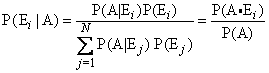The formula in the book is missing the P(Ei) term in the numerator of the center form.  It also had a B term in the denominator which should be A.  Sorry about that.  How embarrassing!

I also added the simpler, equivalent form at the right.  P(A • E) is the same as the numerator, center.  P(A) is the same as the denominator, center.  This is much easier to remember, isn't it?  If you have a joint probability table, conditional probabilities are always the ratio of a joint probability (inside the table) divided by a marginal probability (at the margin of the table).  This is the Bayes' rule method that use in classes.

Page 467, (6.) formula for recoverable reserves per well (2nd edition, 2007 printing and earlier)

The right-hand side is missing the a denominator. The formula should read: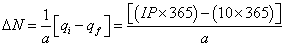#### Page 520, Obsolete reference (2nd edition, 2007 printing and earlier)

About the middle of the page is a reference to a 5-column table method for solving Bayes' theorem. Table 6.17 was in the first edition and deleted from the second edition. The method is shown in the tables on page 323 and 521.

In the last paragraph,

"That is, the column 3 values we need are P(B | E1)and P(B | E1)." should read

"That is, the column 3 values we need are P(B | E1)and P(B | E2).

Page 521, Table has truncated labels

The lower-right annotations should be:

← P(E1 | B)           and

← P(E2 | B)

Page 522, Notation typo

P(E2)  =  0.3

#### Page 564, Figure 11.5, Risk versus value of investment candidates (prior to the 2005 printing).

Perhaps you figured out the different symbols, where I forgot to label the symbols:

Hollow squares represent investments approved, subject to a budget constraint.
Hollow squares plus solid circles represent acceptable projects (better than the rightmost dotted contour, which represents the boundary between acceptable and unacceptable projects).
Hollow circles represent unacceptable projects.

On page 565, the third paragraph, "Figure 11" should be "Figure 11.5."

Please email me to report any problems, small or big, at john1@maxvalue.com   Thanks for reading the book!

revised 11-May-2019